$$\newcommand{\id}{\mathrm{id}}$$ $$\newcommand{\Span}{\mathrm{span}}$$ $$\newcommand{\kernel}{\mathrm{null}\,}$$ $$\newcommand{\range}{\mathrm{range}\,}$$ $$\newcommand{\RealPart}{\mathrm{Re}}$$ $$\newcommand{\ImaginaryPart}{\mathrm{Im}}$$ $$\newcommand{\Argument}{\mathrm{Arg}}$$ $$\newcommand{\norm}{\| #1 \|}$$ $$\newcommand{\inner}{\langle #1, #2 \rangle}$$ $$\newcommand{\Span}{\mathrm{span}}$$

# 5.3: Solve Systems of Equations by Elimination

$$\newcommand{\vecs}{\overset { \rightharpoonup} {\mathbf{#1}} }$$ $$\newcommand{\vecd}{\overset{-\!-\!\rightharpoonup}{\vphantom{a}\smash {#1}}}$$$$\newcommand{\id}{\mathrm{id}}$$ $$\newcommand{\Span}{\mathrm{span}}$$ $$\newcommand{\kernel}{\mathrm{null}\,}$$ $$\newcommand{\range}{\mathrm{range}\,}$$ $$\newcommand{\RealPart}{\mathrm{Re}}$$ $$\newcommand{\ImaginaryPart}{\mathrm{Im}}$$ $$\newcommand{\Argument}{\mathrm{Arg}}$$ $$\newcommand{\norm}{\| #1 \|}$$ $$\newcommand{\inner}{\langle #1, #2 \rangle}$$ $$\newcommand{\Span}{\mathrm{span}}$$ $$\newcommand{\id}{\mathrm{id}}$$ $$\newcommand{\Span}{\mathrm{span}}$$ $$\newcommand{\kernel}{\mathrm{null}\,}$$ $$\newcommand{\range}{\mathrm{range}\,}$$ $$\newcommand{\RealPart}{\mathrm{Re}}$$ $$\newcommand{\ImaginaryPart}{\mathrm{Im}}$$ $$\newcommand{\Argument}{\mathrm{Arg}}$$ $$\newcommand{\norm}{\| #1 \|}$$ $$\newcommand{\inner}{\langle #1, #2 \rangle}$$ $$\newcommand{\Span}{\mathrm{span}}$$

##### Learning Objectives

By the end of this section, you will be able to:

• Solve a system of equations by elimination
• Solve applications of systems of equations by elimination
• Choose the most convenient method to solve a system of linear equations
##### Note

Before you get started, take this readiness quiz.

1. Simplify −5(6−3a).
If you missed this problem, review Exercise 1.10.43.
2. Solve the equation $$\frac{1}{3}x+\frac{5}{8}=\frac{31}{24}$$.
If you missed this problem, review Exercise 2.5.1.

We have solved systems of linear equations by graphing and by substitution. Graphing works well when the variable coefficients are small and the solution has integer values. Substitution works well when we can easily solve one equation for one of the variables and not have too many fractions in the resulting expression.

The third method of solving systems of linear equations is called the Elimination Method. When we solved a system by substitution, we started with two equations and two variables and reduced it to one equation with one variable. This is what we’ll do with the elimination method, too, but we’ll have a different way to get there.

## Solve a System of Equations by Elimination

The Elimination Method is based on the Addition Property of Equality. The Addition Property of Equality says that when you add the same quantity to both sides of an equation, you still have equality. We will extend the Addition Property of Equality to say that when you add equal quantities to both sides of an equation, the results are equal.

For any expressions a, b, c, and d,

$\begin{array}{lc} \text{ if } & a=b \\ \text { and } & c=d \\ \text { then } &a+c =b+d \end{array}$

To solve a system of equations by elimination, we start with both equations in standard form. Then we decide which variable will be easiest to eliminate. How do we decide? We want to have the coefficients of one variable be opposites, so that we can add the equations together and eliminate that variable.

Notice how that works when we add these two equations together:

$\begin{array}{l} 3x+y=5 \\ \underline{2x-y=0} \\ 5x\quad\quad=5\end{array}$

The y’s add to zero and we have one equation with one variable.

Let’s try another one:

$\left\{\begin{array}{l}{x+4 y=2} \\ {2 x+5 y=-2}\end{array}\right.$

This time we don’t see a variable that can be immediately eliminated if we add the equations.

But if we multiply the first equation by −2, we will make the coefficients of x opposites. We must multiply every term on both sides of the equation by −2.Now we see that the coefficients of the x terms are opposites, so x will be eliminated when we add these two equations.

Add the equations yourself—the result should be −3y = −6. And that looks easy to solve, doesn’t it? Here is what it would look like.We’ll do one more:

$\left\{\begin{array}{l}{4 x-3 y=10} \\ {3 x+5 y=-7}\end{array}\right.$

It doesn’t appear that we can get the coefficients of one variable to be opposites by multiplying one of the equations by a constant, unless we use fractions. So instead, we’ll have to multiply both equations by a constant.

We can make the coefficients of x be opposites if we multiply the first equation by 3 and the second by −4, so we get 12x and −12x.This gives us these two new equations:

\left\{\begin{aligned} 12 x-9 y &=30 \\-12 x-20 y &=28 \end{aligned}\right.

$\[\left\{\begin{array}{r}{12 x-9 y=30} \\ {\underline{-12 x-20 y=28}} \\\end{array}\right.\\\quad\qquad {-29 y=58}$\]

the x’s are eliminated and we just have −29y = 58.

Once we get an equation with just one variable, we solve it. Then we substitute that value into one of the original equations to solve for the remaining variable. And, as always, we check our answer to make sure it is a solution to both of the original equations.

Now we’ll see how to use elimination to solve the same system of equations we solved by graphing and by substitution.

##### Exercise $$\PageIndex{1}$$: How to Solve a System of Equations by Elimination

Solve the system by elimination. $$\left\{\begin{array}{l}{2 x+y=7} \\ {x-2 y=6}\end{array}\right.$$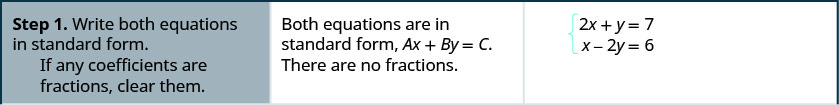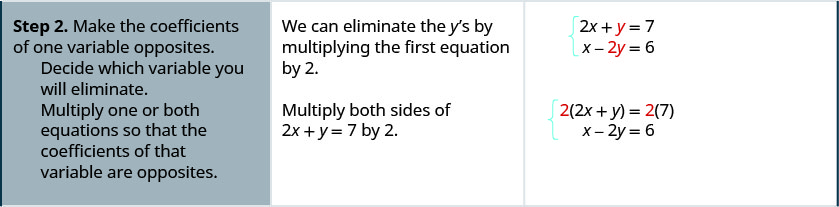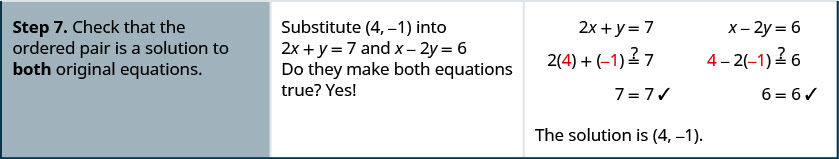##### Exercise $$\PageIndex{2}$$

Solve the system by elimination. $$\left\{\begin{array}{l}{3 x+y=5} \\ {2 x-3 y=7}\end{array}\right.$$

(2,−1)

##### Exercise $$\PageIndex{3}$$

Solve the system by elimination. $$\left\{\begin{array}{l}{4 x+y=-5} \\ {-2 x-2 y=-2}\end{array}\right.$$

(−2,3)

The steps are listed below for easy reference.

##### HOW TO SOLVE A SYSTEM OF EQUATIONS BY ELIMINATION.
1. Write both equations in standard form. If any coefficients are fractions, clear them.
2. Make the coefficients of one variable opposites.
• Decide which variable you will eliminate.
• Multiply one or both equations so that the coefficients of that variable are opposites.
3. Add the equations resulting from Step 2 to eliminate one variable.
4. Solve for the remaining variable.
5. Substitute the solution from Step 4 into one of the original equations. Then solve for the other variable.
6. Write the solution as an ordered pair.
7. Check that the ordered pair is a solution to both original equations.

First we’ll do an example where we can eliminate one variable right away.

##### Exercise $$\PageIndex{4}$$

Solve the system by elimination. $$\left\{\begin{array}{l}{x+y=10} \\ {x-y=12}\end{array}\right.$$Both equations are in standard form. The coefficients of y are already opposites. Add the two equations to eliminate y. The resulting equation has only 1 variable, x.Solve for x, the remaining variable. Substitute x = 11 into one of the original equations.Solve for the other variable, y.Write the solution as an ordered pair. The ordered pair is (11, −1). Check that the ordered pair is a solution to both original equations. $$\begin{array}{rllrll} x+y &=&10 &x-y&=&12\\ 11+(-1) &\stackrel{?}{=}&10 & 11-(-1) &\stackrel{?}{=}&12\\ 10 &=&10 \checkmark & 12 &=&12 \checkmark \end{array}$$ The solution is (11, −1).
##### Exercise $$\PageIndex{5}$$

Solve the system by elimination. $$\left\{\begin{array}{l}{2 x+y=5} \\ {x-y=4}\end{array}\right.$$

(3,−1)

##### Exercise $$\PageIndex{6}$$

Solve the system by elimination.$$\left\{\begin{array}{l}{x+y=3} \\ {-2 x-y=-1}\end{array}\right.$$

(−2,5)

In Exercise $$\PageIndex{7}$$, we will be able to make the coefficients of one variable opposites by multiplying one equation by a constant.

##### Exercise $$\PageIndex{7}$$

Solve the system by elimination. $$\left\{\begin{array}{l}{3 x-2 y=-2} \\ {5 x-6 y=10}\end{array}\right.$$Both equations are in standard form. None of the coefficients are opposites. We can make the coefficients of y opposites by multiplying the first equation by −3.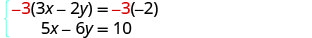Simplify.Add the two equations to eliminate y.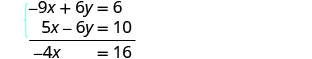Solve for the remaining variable, x. Substitute x = −4 into one of the original equations.Solve for y.Write the solution as an ordered pair. The ordered pair is (−4, −5). Check that the ordered pair is a solution to both original equations. $$\begin{array}{rllrll} 3x-2y &=&-2 &5x-6y&=&10\\ 3(-4)-2(-5) &\stackrel{?}{=}&-2 & 5(-4)-6(-5) &\stackrel{?}{=}&10\\ -12+10&\stackrel{?}{=}&-2 &-20+30&\stackrel{?}{=}&10\\-2 &=&-2 \checkmark & 10 &=&10 \checkmark \end{array}$$ The solution is (−4, −5).
##### Exercise $$\PageIndex{8}$$

Solve the system by elimination.$$\left\{\begin{array}{l}{4 x-3 y=1} \\ {5 x-9 y=-4}\end{array}\right.$$

(1,1)

##### Exercise $$\PageIndex{9}$$

Solve the system by elimination.$$\left\{\begin{array}{l}{3 x+2 y=2} \\ {6 x+5 y=8}\end{array}\right.$$

(−2,4)

Now we’ll do an example where we need to multiply both equations by constants in order to make the coefficients of one variable opposites.

##### Exercise $$\PageIndex{10}$$

Solve the system by elimination. $$\left\{\begin{array}{l}{4 x-3 y=9} \\ {7 x+2 y=-6}\end{array}\right.$$

In this example, we cannot multiply just one equation by any constant to get opposite coefficients. So we will strategically multiply both equations by a constant to get the opposites.Both equations are in standard form. To get opposite coefficients of y, we will multiply the first equation by 2 and the second equation by 3.Simplify.Add the two equations to eliminate y.Solve for x. Substitute x = 0 into one of the original equations.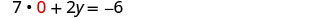Solve for y.Write the solution as an ordered pair. The ordered pair is (0, −3). Check that the ordered pair is a solution to both original equations. $$\begin{array}{rllrll} 4x-3y &=&9 &7x+2y&=&-6\\ 4(0)-3(-3) &\stackrel{?}{=}&9 & 7(0)+2(-3) &\stackrel{?}{=}&-6\\9 &=&9 \checkmark & -6 &=&-6 \checkmark \end{array}$$ The solution is (0, −3).
##### Exercise $$\PageIndex{11}$$

Solve the system by elimination. $$\left\{\begin{array}{l}{3 x-4 y=-9} \\ {5 x+3 y=14}\end{array}\right.$$

(1,3)

##### Exercise $$\PageIndex{12}$$

Solve the system by elimination. $$\left\{\begin{array}{l}{7 x+8 y=4} \\ {3 x-5 y=27}\end{array}\right.$$

(4,−3)

When the system of equations contains fractions, we will first clear the fractions by multiplying each equation by its LCD.

##### Exercise $$\PageIndex{13}$$

Solve the system by elimination. $$\left\{\begin{array}{l}{x+\frac{1}{2} y=6} \\ {\frac{3}{2} x+\frac{2}{3} y=\frac{17}{2}}\end{array}\right.$$

In this example, both equations have fractions. Our first step will be to multiply each equation by its LCD to clear the fractions.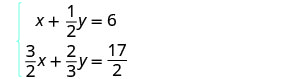To clear the fractions, multiply each equation by its LCD.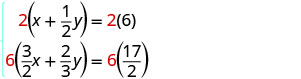Simplify.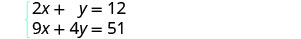Now we are ready to eliminate one of the variables. Notice that both equations are in standard form. We can eliminate y multiplying the top equation by −4.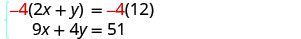Simplify and add. Substitute x = 3 into one of the original equations.Solve for y.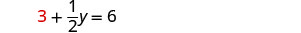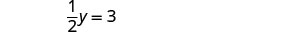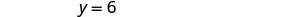Write the solution as an ordered pair. The ordered pair is (3, 6). Check that the ordered pair is a solution to both original equations. $$\begin{array}{rllrll} x+\frac{1}{2}y &=&6 &\frac{3}{2}x+\frac{2}{3}y&=&\frac{17}{2}\\ 3+\frac{1}{2}(6) &\stackrel{?}{=}&6 &\frac{3}{2}(3) + \frac{2}{3}(6)&\stackrel{?}{=}&\frac{17}{2}\\ 3 + 3 &\stackrel{?}{=}&6 & \frac{9}{2 }+4 &\stackrel{?}{=} & \frac{17}{2}\\ 6 &=&6 \checkmark & \frac{9}{2} + \frac{8}{2} &\stackrel{?}{=} & \frac{17}{2}\\ && & \frac{17}{2} &=&\frac{17}{2} \checkmark \end{array}$$ The solution is (3, 6).
##### Exercise $$\PageIndex{14}$$

Solve the system by elimination. $$\left\{\begin{array}{l}{\frac{1}{3} x-\frac{1}{2} y=1} \\ {\frac{3}{4} x-y=\frac{5}{2}}\end{array}\right.$$

(6,2)

##### Exercise $$\PageIndex{15}$$

Solve the system by elimination. $$\left\{\begin{array}{l}{x+\frac{3}{5} y=-\frac{1}{5}} \\ {-\frac{1}{2} x-\frac{2}{3} y=\frac{5}{6}}\end{array}\right.$$

(1,−2)

In the Solving Systems of Equations by Graphing we saw that not all systems of linear equations have a single ordered pair as a solution. When the two equations were really the same line, there were infinitely many solutions. We called that a consistent system. When the two equations described parallel lines, there was no solution. We called that an inconsistent system.

##### Exercise $$\PageIndex{16}$$

Solve the system by elimination.$$\left\{\begin{array}{l}{3 x+4 y=12} \\ {y=3-\frac{3}{4} x}\end{array}\right.$$

\begin{array} {ll} & \left\{\begin{aligned} 3 x+4 y &=12 \\ y &=3-\frac{3}{4} x \end{aligned}\right. \\\\\text{Write the second equation in standard form.} & \left\{\begin{array}{l}{3 x+4 y=12} \\ {\frac{3}{4} x+y=3}\end{array}\right.\\ \\ \text{Clear the fractions by multiplying thesecond equation by 4.} & \left\{\begin{aligned} 3 x+4 y &=12 \\ 4\left(\frac{3}{4} x+y\right) &=4(3) \end{aligned}\right. \\\\ \text{Simplify.} & \left\{\begin{array}{l}{3 x+4 y=12} \\ {3 x+4 y=12}\end{array}\right.\\\\ \text{To eliminate a variable, we multiply thesecond equation by −1.} & \left\{\begin{array}{c}{3 x+4 y=12} \\ \underline{-3 x-4 y=-12} \end{array}\right.\\ &\qquad\qquad\quad 0=0 \\ \text{Simplify and add.} \end{array}

This is a true statement. The equations are consistent but dependent. Their graphs would be the same line. The system has infinitely many solutions.

After we cleared the fractions in the second equation, did you notice that the two equations were the same? That means we have coincident lines.

##### Exercise $$\PageIndex{17}$$

Solve the system by elimination. $$\left\{\begin{array}{l}{5 x-3 y=15} \\ {y=-5+\frac{5}{3} x}\end{array}\right.$$

infinitely many solutions

##### Exercise $$\PageIndex{18}$$

Solve the system by elimination. $$\left\{\begin{array}{l}{x+2 y=6} \\ {y=-\frac{1}{2} x+3}\end{array}\right.$$

infinitely many solutions

##### Exercise $$\PageIndex{19}$$

Solve the system by elimination. $$\left\{\begin{array}{l}{-6 x+15 y=10} \\ {2 x-5 y=-5}\end{array}\right.$$

\begin{array} {ll} \text{The equations are in standard form.}& \left\{\begin{aligned}-6 x+15 y &=10 \\ 2 x-5 y &=-5 \end{aligned}\right. \\\\ \text{Multiply the second equation by 3 to eliminate a variable.} & \left\{\begin{array}{l}{-6 x+15 y=10} \\ {3(2 x-5 y)=3(-5)}\end{array}\right. \\\\ \text{Simplify and add.} & \left\{\begin{aligned}{-6 x+15 y =10} \\ \underline{6 x-15 y =-15} \end{aligned}\right. \\ & \qquad \qquad \quad0\neq 5 \end{array}

This statement is false. The equations are inconsistent and so their graphs would be parallel lines.

The system does not have a solution.

##### Exercise $$\PageIndex{20}$$

Solve the system by elimination. $$\left\{\begin{array}{l}{-3 x+2 y=8} \\ {9 x-6 y=13}\end{array}\right.$$

no solution

##### Exercise $$\PageIndex{21}$$

Solve the system by elimination. $$\left\{\begin{array}{l}{7 x-3 y=-2} \\ {-14 x+6 y=8}\end{array}\right.$$

no solution

## Solve Applications of Systems of Equations by Elimination

Some applications problems translate directly into equations in standard form, so we will use the elimination method to solve them. As before, we use our Problem Solving Strategy to help us stay focused and organized.

##### Exercise $$\PageIndex{22}$$

The sum of two numbers is 39. Their difference is 9. Find the numbers.

$$\begin{array} {ll} \textbf{Step 1. Read}\text{ the problem}& \\ \textbf{Step 2. Identify} \text{ what we are looking for.} & \text{We are looking for two numbers.} \\\textbf{Step 3. Name} \text{ what we are looking for.} & \text{Let n = the first number.} \\ & \text{ m = the second number} \\\textbf{Step 4. Translate} \text{ into a system of equations.}& \\ & \text{The sum of two numbers is 39.} \\ & n+m=39\\ & \text{Their difference is 9.} \\ & n−m=9 \\ \\ \text{The system is:} & \left\{\begin{array}{l}{n+m=39} \\ {n-m=9}\end{array}\right. \\\\ \textbf{Step 5. Solve} \text{ the system of equations. } & \\ \text{To solve the system of equations, use} \\ \text{elimination. The equations are in standard} \\ \text{form and the coefficients of m are} & \\ \text{opposites. Add.} & \left\{\begin{array}{l}{n+m=39} \\ \underline{n-m=9}\end{array}\right. \\ &\quad 2n\qquad=48 \\ \\\text{Solve for n.} & n=24 \\ \\ \text{Substitute n=24 into one of the original} &n+m=39 \\ \text{equations and solve form.} & 24+m=39 \\ & m=15 \\ \textbf{Step 6. Check}\text{ the answer.} & \text{Since 24+15=39 and 24−15=9, the answers check.}\\ \textbf{Step 7. Answer} \text{ the question.} & \text{The numbers are 24 and 15.} \end{array}$$

##### Exercise $$\PageIndex{23}$$

The sum of two numbers is 42. Their difference is 8. Find the numbers.

The numbers are 25 and 17.

##### Exercise $$\PageIndex{24}$$

The sum of two numbers is −15. Their difference is −35. Find the numbers.

The numbers are −25 and 10.

##### Exercise $$\PageIndex{25}$$

Joe stops at a burger restaurant every day on his way to work. Monday he had one order of medium fries and two small sodas, which had a total of 620 calories. Tuesday he had two orders of medium fries and one small soda, for a total of 820 calories. How many calories are there in one order of medium fries? How many calories in one small soda?

 Step 1. Read the problem. Step 2. Identify what we are looking for. We are looking for the number of calories in one order of medium fries and in one small soda. Step 3. Name what we are looking for. Let f = the number of calories in 1 order of medium fries.   s = the number of calories in 1 small soda. Step 4. Translate into a system of equations: one medium fries and two small sodas had a total of 620 caloriestwo medium fries and one small soda had a total of 820 calories.Our system is:Step 5. Solve the system of equations. To solve the system of equations, use elimination. The equations are in standard form. To get opposite coefficients of f, multiply the top equation by −2.Simplify and add.Solve for s.Substitute s = 140 into one of the original equations and then solve for f.Step 6. Check the answer. Verify that these numbers make sense in the problem and that they are solutions to both equations. We leave this to you! Step 7. Answer the question. The small soda has 140 calories and the fries have 340 calories.
##### Exercise $$\PageIndex{26}$$

Malik stops at the grocery store to buy a bag of diapers and 2 cans of formula. He spends a total of $37. The next week he stops and buys 2 bags of diapers and 5 cans of formula for a total of$87. How much does a bag of diapers cost? How much is one can of formula?

The bag of diapers costs $11 and the can of formula costs$13.

##### Exercise $$\PageIndex{27}$$

To get her daily intake of fruit for the day, Sasha eats a banana and 8 strawberries on Wednesday for a calorie count of 145. On the following Wednesday, she eats two bananas and 5 strawberries for a total of 235 calories for the fruit. How many calories are there in a banana? How many calories are in a strawberry?

There are 105 calories in a banana and 5 calories in a strawberry.

## Choose the Most Convenient Method to Solve a System of Linear Equations

When you will have to solve a system of linear equations in a later math class, you will usually not be told which method to use. You will need to make that decision yourself. So you’ll want to choose the method that is easiest to do and minimizes your chance of making mistakes.##### Exercise $$\PageIndex{28}$$

For each system of linear equations decide whether it would be more convenient to solve it by substitution or elimination. Explain your answer.

1. $$\left\{\begin{array}{l}{3 x+8 y=40} \\ {7 x-4 y=-32}\end{array}\right.$$
2. $$\left\{\begin{array}{l}{5 x+6 y=12} \\ {y=\frac{2}{3} x-1}\end{array}\right.$$

1. $$\left\{\begin{array}{l}{3 x+8 y=40} \\ {7 x-4 y=-32}\end{array}\right.$$

Since both equations are in standard form, using elimination will be most convenient.

2. $$\left\{\begin{array}{l}{5 x+6 y=12} \\ {y=\frac{2}{3} x-1}\end{array}\right.$$

Since one equation is already solved for y, using substitution will be most convenient.

##### Exercise $$\PageIndex{29}$$

For each system of linear equations, decide whether it would be more convenient to solve it by substitution or elimination. Explain your answer.

1. $$\left\{\begin{array}{l}{4 x-5 y=-32} \\ {3 x+2 y=-1}\end{array}\right.$$
2. $$\left\{\begin{array}{l}{x=2 y-1} \\ {3 x-5 y=-7}\end{array}\right.$$
1. Since both equations are in standard form, using elimination will be most convenient.
2. Since one equation is already solved for xx, using substitution will be most convenient.
##### Exercise $$\PageIndex{30}$$

For each system of linear equations, decide whether it would be more convenient to solve it by substitution or elimination. Explain your answer.

1. $$\left\{\begin{array}{l}{y=2 x-1} \\ {3 x-4 y=-6}\end{array}\right.$$
2. $$\left\{\begin{array}{l}{6 x-2 y=12} \\ {3 x+7 y=-13}\end{array}\right.$$
1. Since one equation is already solved for yy, using substitution will be most convenient;
2. Since both equations are in standard form, using elimination will be most convenient.
##### Note

Access these online resources for additional instruction and practice with solving systems of linear equations by elimination.

## Key Concepts

• To Solve a System of Equations by Elimination
1. Write both equations in standard form. If any coefficients are fractions, clear them.
2. Make the coefficients of one variable opposites.
• Decide which variable you will eliminate.
• Multiply one or both equations so that the coefficients of that variable are opposites.
3. Add the equations resulting from Step 2 to eliminate one variable.
4. Solve for the remaining variable.
5. Substitute the solution from Step 4 into one of the original equations. Then solve for the other variable.
6. Write the solution as an ordered pair.
7. Check that the ordered pair is a solution to both original equations.